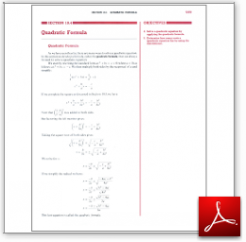Algebra Math Book - Introductory Algebra Thank you for your continual support.

 The power of mathematics rests in the logic of thinking.ID: Sec10-4
Description: Development of the Quadratic Formula-The Discriminant
Price: 1.99

# Algebra Math book - Introductory Algebra - Chapter 10 - Section 4 - Quadratic Formula

## Section 10.4 - Objectives

5.  Determine how many roots a quadratic equation has using the discriminant.

Navigate to
Previous Section or Next Section
or Chapter 10 Details or Other Chapters

This section of my algebra math book, Introductory Algebra, also includes in the download:

• Cover Sheet